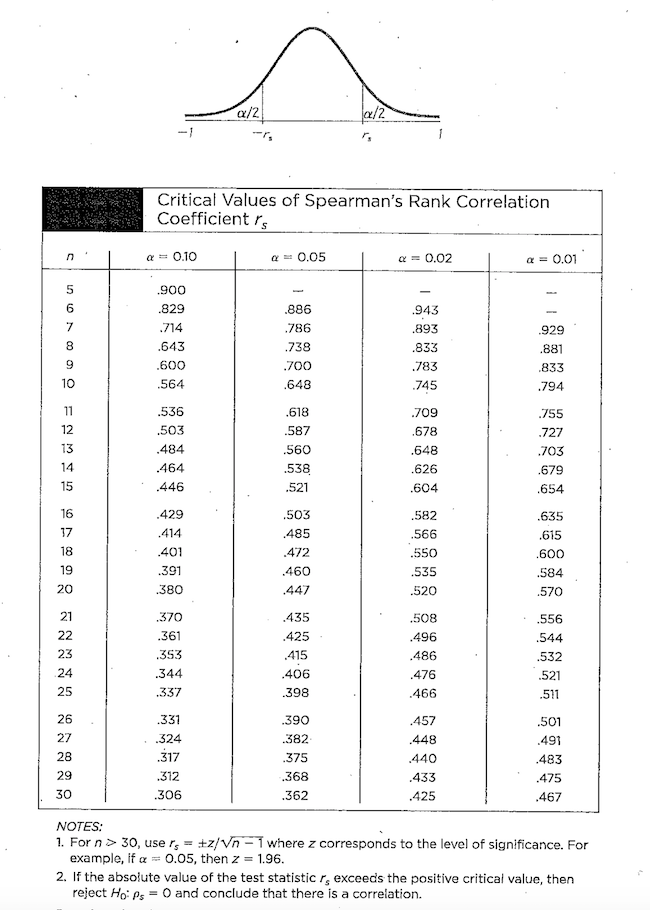## Spearman's Rank Sum Correlation Test

There is a non-parametric test for an association (not necessarily linear) between two variables, called Spearman's Rank Correlation Test that can be used when the assumptions/requirements of the (parametric) correlation test are not satisfied.

The only requirements of this non-parametric test are that the data is paired and the result of a simple random sample, and that the data can be ranked (if they are not ranks already).

Essentially, all this test does is find ranks $x_i$ and $y_i$ for each pair of $X_i$ and $Y_i$ values and then run Pearson's correlation test on these ranks.

Recall that $$r = \frac{s_{xy}}{s_x s_y} = \frac{\sum_i (x_i - \overline{x})(y_i - \overline{y})}{\sqrt{\sum_i (x_i-\overline{x})^2} \sqrt{\sum_i (y_i - \overline{y})^2}}$$

We denote this value as $r_S$ when it is computed from ranks to avoid confusion.

Procedurally, one ranks each sample separately. Then for each pair, one finds the difference of ranks $d_i$.

The test statistic $r_S$, when there are no rank ties, can be simplified to

$$r_S = 1 - \frac{6 \sum d_i^2}{n(n^2-1)}$$

To see this, first note that as there are no ties, the $x_i$'s and $y_i$'s both consist of the integers from $1$ to $n$, inclusive.

Consequently, we can rewrite the denominator as $$\frac{\sum_i (x_i - \overline{x})(y_i - \overline{y})}{\sum_i (x_i-\overline{x})^2}$$ Ultimately, the denominator is just a function of $n$: $$\begin{array}{rcl} \displaystyle{\sum_{i=1}^n (x_i-\overline{x})^2} & = & \displaystyle{\sum_{i=1}^n x_i^2 - 2\sum_{i=1}^n x_i\overline{x} + \sum_{i=1}^n \overline{x}^2}\\ & = & \displaystyle{\left[ \sum_{i=1}^n x_i^2 \right] - 2n\overline{x}\left[\frac{\sum_{i=1}^n x_i}{n}\right] + n \overline{x}^2}\\ & = & \displaystyle{\left[ \sum_{i=1}^n i^2 \right] - 2n\overline{x}^2 + n \overline{x}^2}\\ & = & \displaystyle{\left[ \sum_{i=1}^n i^2 \right] - n\overline{x}^2}\\ & = & \displaystyle{\frac{n(n+1)(2n+1)}{6} - n \left( \frac{n+1}{2} \right)^2}\\ & = & \displaystyle{n(n+1) \left( \frac{2n+1}{6} - \frac{n+1}{4} \right)}\\ & = & \displaystyle{n(n+1) \left( \frac{8n+4}{24} - \frac{6n+6}{24} \right)}\\ & = & \displaystyle{n(n+1) \left( \frac{2n-2}{24} \right)}\\ & = & \displaystyle{\frac{n(n+1)(n-1)}{12}}\\ & = & \displaystyle{\frac{n(n^2-1)}{12}}\\ \end{array}$$

As for the numerator...

$$\begin{array}{rcl} \displaystyle{\sum_{i=1}^n (x_i - \overline{x})(y_i - \overline{y})} & = & \displaystyle{\sum_{i=1}^n x_i(y_i-\overline{y}) - \sum_{i=1}^n \overline{x} (y_i - \overline{y})}\\ & = & \displaystyle{\sum_{i=1}^n x_i y_i - \overline{y} \sum_{i=1}^n x_i - \overline{x} \sum_{i=1}^n y_i + n \overline{x}\overline{y}}\\ & = & \displaystyle{\left[ \sum_{i=1}^n x_i y_i \right] - n\overline{x}\overline{y}}\\ & = & \displaystyle{\left[ \sum_{i=1}^n x_i y_i \right] - n \left( \frac{n+1}{2} \right)^2}\\ & = & \displaystyle{\left[ \sum_{i=1}^n x_i y_i \right] - \frac{n(n+1)(2n+1)}{6} + \frac{n(n^2-1)}{12}}\\ & = & \displaystyle{\left[ \sum_{i=1}^n x_i y_i \right] - \sum_{i=1}^n x_i^2 + \frac{n(n^2-1)}{12}}\\ & = & \displaystyle{\frac{2\sum_{i=1}^n x_i y_i}{2} - \frac{\sum_{i=1}^n (x_i^2 + y_i^2)}{2} + \frac{n(n^2-1)}{12}}\\ & = & \displaystyle{\frac{n(n^2-1)}{12} - \frac{\sum_{i=1}^n (x_i^2 - 2x_iy_i + y_i^2)}{2}}\\ & = & \displaystyle{\frac{n(n^2-1)}{12} - \frac{\sum_{i=1}^n (x_i - y_i)^2}{2}}\\ & = & \displaystyle{\frac{n(n^2-1)}{12} - \frac{\sum_{i=1}^n d_i^2}{2}}\\ \end{array}$$

Finally, dividing both numerator and denominator by $n(n^2-1)/12$, we can simplify things to

$$r_s = \frac{\displaystyle{\frac{n(n^2-1)}{12} - \frac{\sum_{i=1}^n d_i^2}{2}}}{\displaystyle{\frac{n(n^2-1)}{12}}} = 1 - \frac{6 \sum d_i^2}{n(n^2-1)}$$

Critical values can be found in the table below:Example

Suppose one wishes to use a non-parametric test to test the claim that there is a correlation between one's age and the number of parties they attend in a two-month period, given the following data:

$$\begin{array}{l|c|c|c|c|c|c|c} \textrm{Age} & 16 & 24 & 18 & 17 & 23 & 27 & 32\\\hline \textrm{Parties} & 3 & 2 & 5 & 4 & 0 & 6 & 1 \end{array}$$

First we rank the $x$'s and $y$'s separately:

$$\begin{array}{l|c|c|c|c|c|c|c} & 1 & 5 & 3 & 2 & 4 & 6 & 7 \\\hline \textrm{Age} & 16 & 24 & 18 & 17 & 23 & 27 & 32\\\hline \textrm{Parties} & 3 & 2 & 5 & 4 & 0 & 6 & 1\\\hline & 4 & 3 & 6 & 5 & 1 & 7 & 2 \end{array}$$

Then, for each pair, we find the difference of the ranks and its square.

$$\begin{array}{l|c|c|c|c|c|c|c} d & -3 & 2 & -3 & -3 & 3 & -1 & 5\\\hline d^2 & 9 & 4 & 9 & 9 & 9 & 1 & 25 \end{array}$$

Now we can calculate the test statistic:

$$r_S = 1 - \frac{6 \sum d_i^2}{n(n^2-1)} = 1 - \frac{(6)(66)}{(7)(49-1)} = -0.1786$$

Seeing this test statistic less in absolute value than the corresponding critical value at $\alpha = 0.05$ given in the table above (i.e., $C.V. = 0.786$), we would fail to reject the null hypothesis, inferring that there is no evidence of a correlation.